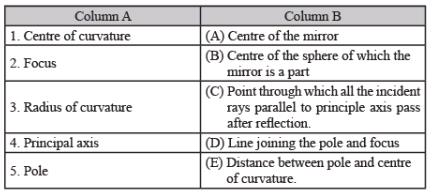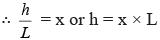Courses

# Olympiad Test: Light - 2

## 10 Questions MCQ Test Science Olympiad Class 7 | Olympiad Test: Light - 2

Description
This mock test of Olympiad Test: Light - 2 for Class 7 helps you for every Class 7 entrance exam. This contains 10 Multiple Choice Questions for Class 7 Olympiad Test: Light - 2 (mcq) to study with solutions a complete question bank. The solved questions answers in this Olympiad Test: Light - 2 quiz give you a good mix of easy questions and tough questions. Class 7 students definitely take this Olympiad Test: Light - 2 exercise for a better result in the exam. You can find other Olympiad Test: Light - 2 extra questions, long questions & short questions for Class 7 on EduRev as well by searching above.
QUESTION: 1

Solution:
QUESTION: 2

Solution:
QUESTION: 3

### Radius of curvature for a plane mirror is

Solution:
QUESTION: 4

The distance between the focus and the pole of the mirror is called

Solution:
QUESTION: 5

In case of mirror match the columns A with columns B:Solution:
QUESTION: 6

At a particular time of a day, the ratio of height of an object and the length of it’s shadow is ‘x’. Using this calculate the height of a tree if the length of the shadow of tree is L.

Solution:

During the same time the ratio of the height and length of any object will be equal to that of any other including ‘x’.
Step 1- Let the height of the tree be ‘h’. The length of the tree is given as ‘L’
∴ the ratio of its height and length would be h/L
Step 2- This ratio will be the same as ‘x’QUESTION: 7

Prism has

Solution:
QUESTION: 8

A series of fast moving still pictures can create an illusion of movement because

Solution:

This happens due to a phenomenon called persistence of vision.

QUESTION: 9

In a periscope, the reflecting mirror will be

Solution:
QUESTION: 10

Periscope is used to

Solution: